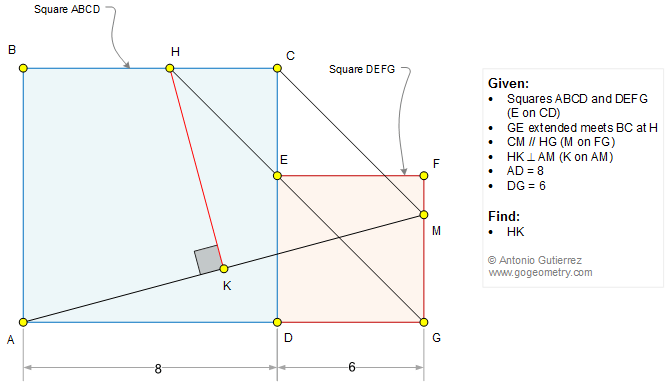# Geometry Problem 1331: Two Squares Side by Side, Parallel, Perpendicular, 90 Degrees, Measurement. Level: High School. Math Infographic.

< PREVIOUS PROBLEM  |  NEXT PROBLEM >

 The figure below shows the squares ABCD and DEFG (E on CD). GE extended meets BC at H. CM is parallel to HG (M on FG) and HK is perpendicular to AM (K on AM). If AD = 8 and DG = 6, find the measure of HK.Home | Search | Geometry | Problems | All Problems | Open Problems | Visual Index | 10 Problems | Ten problems: 1331-1340 | Triangle | Square | Parallel lines | Perpendicular line | 45 degrees | Congruence | View or Post a solution | by Antonio Gutierrez